# Delta Neutral | The 5 Most Popular Options Trading Strategies

## When hedging options, delta neutral strategies are some of the most popular. A delta neutral position allows traders to profit no matter which direction the underlying asset moves. Many different delta neutral strategies can be used for a delta neutral portfolio, but in this blog post, we will focus on the five most popular ones.## Basic Delta Value Explained

To understand delta neutral position trading, it is crucial to have a basic understanding of the delta value. Delta measures how much the price of an option position will change with changes in the underlying asset prices.

In a scenario where a call option has a position delta value of 0.50, that means that for every \$1, the underlying security increases in price, the call option will increase by \$0.50. Alternatively, if a stock position delta value of a put option is -0.50, that means that for every \$1, the underlying security decreases in price, the put options will increase by \$0.50.

It is also important to note that call option delta values can range from 0.0 to + 100.0. A delta value of 0.0 means that the option will not change with the underlying security's price, while a put option delta value ranges from 0.0 to - 100.00. A positive or negative one hundred is the maximum value delta can ever reach because every option controls one hundred shares of the underlying asset.

## What is Delta Neutral Options Trading?

Now that we understand the value of positive and negative deltas, we can move on to delta neutral hedging. Delta neutral options trading is when you buy and sell options so that your overall delta value is zero.

In such a scenario where you are bullish on stock XYZ and believe it will increase the price over the next few weeks. You could buy a call option with a delta value of 0.50, and to offset the delta, you would sell two call options with delta values of -0.25 each. This would give you an overall delta value of zero because 0.50 + (-0.25) + (-0.25) = 0.

While the stock moves the overall portfolio delta value will change which means traders have to constantly adjust delta daily, weekly, or monthly. Strategies to adjust delta will be talked about later in this article.

## How do you calculate delta neutral positions?

To calculate a delta neutral portfolio, you need to take the delta value of all the options you are long and add them together. Then, take the delta value of all the options you are short on and subtract them. The difference between these two values will be your overall delta.

If your overall delta is not zero, you can adjust your position by buying or selling options until it is. In the picture below, our portfolio is short and long many options for Facebook.

After adding and subtracting all the deltas from the options, we see that the net delta is +10. If this portfolio targets a delta neutral position, the trader would need to use the trading strategies below to reduce it. Being delta neutral is ideal if the trader believes the market will trade sideways.## Benefits of Delta Neutral Trading

Many benefits come with delta neutral trading. First and foremost, it allows traders to profit no matter which direction the underlying security moves. If you are delta neutral and the underlying stock price goes up, you will profit from your long call option. If the underlying asset price goes down, you will profit from your short-call options.

Another benefit of delta neutral trading is that it can help you avoid theta decay. Theta is the amount by which an option's value declines over time. When you are delta neutral, you are effectively buying and selling options simultaneously, which can help offset the effects of theta decay.

Finally, delta neutral trading can also help to reduce your overall potential loss. When you are delta neutral, you are not placing all of your eggs in one basket. If the trade does not go as planned, you will still have a chance to make money off of the other side of the trade.

## Does Delta Neutral Trading Work With the Underlying Asset?

A delta neutral trading can be an effective way to profit from the markets. However, it is essential to remember that no portfolio strategy is perfect, and risks are always involved. Before implementing any delta neutral strategy, do your research and understand all potential risks and rewards.

One thing to keep in mind with this delta neutral strategy is that it only works if the underlying security's price does not move much. If the underlying asset goes up or down significantly, you could lose money.

## Example of a Delta Neutral Position

To go in-depth on many popular delta neutral strategies, we need to pick an underlying asset to theoretically hedge with delta. The security used in this example will be 200 shares of Tilray stock trading at \$8.17. This position gives us a total investment of \$1,634 and a delta position of +200.

A delta position of +200 means for every dollar move of the stock higher, our account will increase by \$200, and for every dollar move of the underlying stock lower, our account will decrease by \$200.

These types of account swings may not be comfortable for a trader to stomach, and this is where delta hedging or delta neutral strategies can help!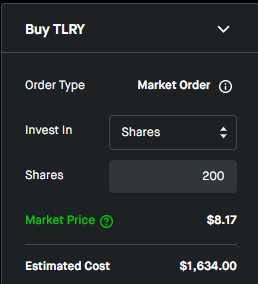## #1 Delta Neutral Strategy | Covered Calls

The covered call is a delta neutral strategy that involves selling one call option against every 100 shares of the underlying security.

For example, if we sold two Tilray calls with a strike of \$10 expiring in 5 days, we would be closer to delta neutral. The trade would look like this:

• We are long 200 shares which gives a delta of +200
• We short two call options with a strike of \$10 and an expiration date of 4/1, which has a delta of -76
• Our net delta position is +128
• Possible credit from the short options is +\$134

This new delta of +128 means that our potential loss is lower, but our position is still long because of the significant moves in the delta.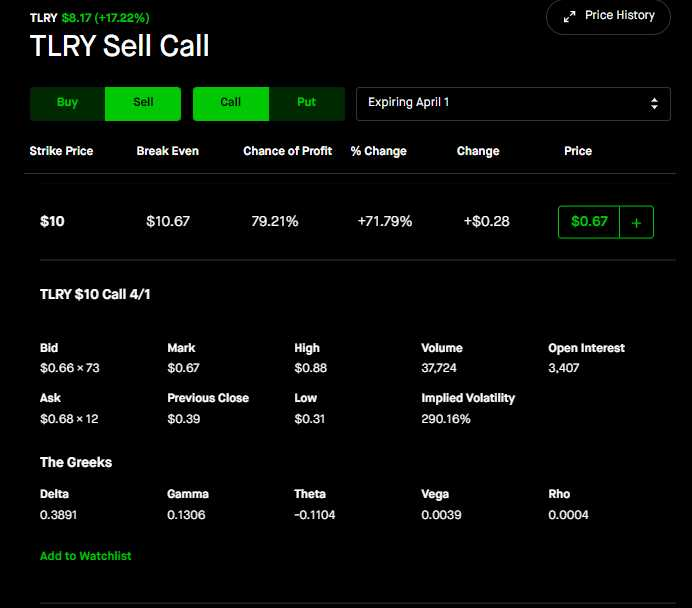Our example of the \$10 strike price does not imply this is the best option to use to hedge for the trader. The trader can use different strike prices or expiration dates. To further illustrate this, we will give examples below:

• Selling 2 Call Options with a strike price of \$4 will provide a delta of -198
• Selling 2 Call Options with a strike price of \$7 will give a delta of -186
• Selling 2 Call Options with a strike price of \$8.5 will provide a delta of -122
• Selling 2 Call Options with a strike price of \$9.5 will provide a delta of -8

As the trader increases the call options strike price, less of the initial investment is being hedged because of the decrease of the delta. Therefore, the best options to hedge all of the delta will be found deep in the money instead of out of the money.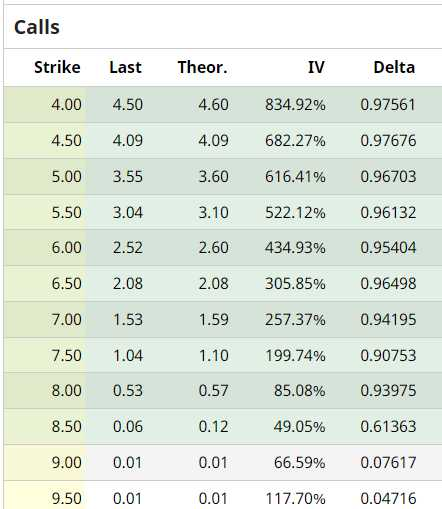### Inability to Fully Hedge

While this is a delta neutral strategy, it doesn't mean this strategy alone can produce a delta neutral position. Most option traders will need to combine multiple other delta neutral strategies to achieve delta neutrality.

### Possible Margin Call

The other choice is for the trader to use margin to short more call options to achieve neutrality. Using margin is not suggested as it can increase the potential loss for the trader if the underlying stock moves past the strike prices shorted and place the trader in a margin call. That is why it is important to use other delta heading strategies that don't dramatically increase the risk for the trader.

Unfortunately, this will need to be done for the example above. So we will look to the next delta neutral strategy to further reduce our delta.

## #2 Delta Neutral Strategy | Long Puts or Calls

The long put delta neutral strategy is the opposite of the covered call delta neutral strategy.

Instead of selling calls against our long underlying stock position, we will buy puts to help us achieve delta neutrality.

Going back to our original Tilray example above, our net delta is still +128. Any long put option will have a negative delta allowing the trader to lower their net delta position.

To decrease of delta even further, the trader could:

• Buy 3 Tilray Put Options for the Strike \$8.5 and an expiration date of 4/1, which would give a -129 delta (shown in the example below)
• Given our previous +128 delta, this new purchase reduces our delta to -1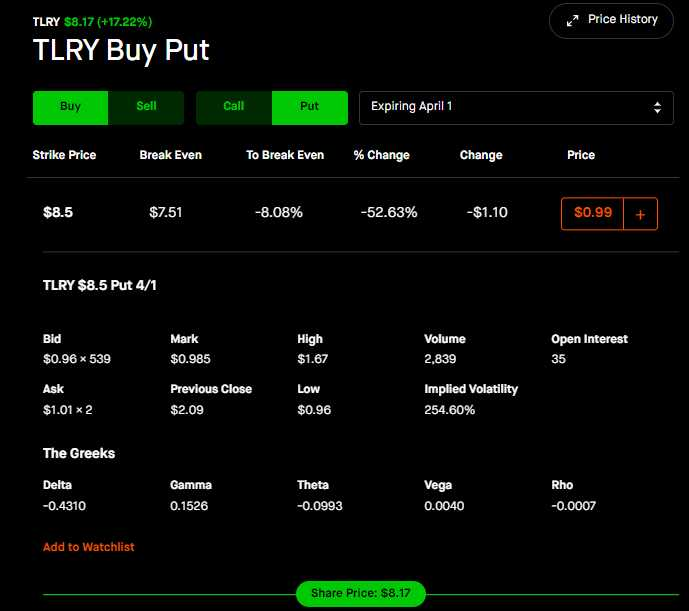While net delta is now achieved, the trader needs to be aware that the credit and cost from the covered call (+\$134) and long put (-\$300) are putting +\$164 at risk now.

This means that the act of achieving delta neutral is putting money at risk. There is no right way to hedge delta neutral portfolio, and this choice is up to the trader to make and fully understand. To further illustrate what combinations the trader could have chosen, we will look at different strike prices and expiration dates below.

This neutrality could have also been achieved by:

• Buying 2 Puts with a strike price of \$10 and with Delta -60 would give a total delta of -120
• Buying 5 Puts with a strike price of \$7.5 and with Delta -26 would give a total delta of -130
• Buying 3 Puts with expiration three months further out for the same strike price would give a total delta of -130

### The downside of hedging with long options

There are some significant downsides to hedging with long options that every trader needs to know.

• Increased time decay - using this strategy will put the trader at risk of losing money every day from holding these long options
• Increased capital at risk - using this strategy costs money and means the trader can lose all the capital invested into the long option

## #3 Delta Neutral Strategy | Credit or Debit Spreads

The next way to lower or raise delta is through credit spreads. Credit spreads can allow traders also to lower theta or vega, which is beneficial, and we will discuss this later in the article.

An option spread is created from buying and selling an option simultaneously. The difference between those strike prices for the call or put options can give the trader a way to offset delta, theta, or vega.

Below we will give four examples of hedging an options position with a credit or debit spread:

• Call Debit spread: In the example below, you can see on the right side of the screen all the deltas are positive. The trader can increase the delta by buying a call, debit spread.
• Call Credit spread: The trader can also achieve the exact opposite by shorting the spread, which would create a call credit spread. By selling a call credit spread, the trader can decrease the delta. For example, previously, with a delta of +128, the trader would need to short roughly 15-30 contracts to achieve neutrality.
• Put debit spread: In the example below, you can see on the left side of the screen all the deltas are negative. By buying a put debit spread, the trader can decrease the delta. For example, previously, with a delta of +128, the trader would need to buy long, roughly 15-30 contracts to achieve neutrality.
• Put credit spread: The trader can also achieve the exact opposite by shorting the spread, which would create a put credit spread. By selling a put credit spread, the trader can increase the delta.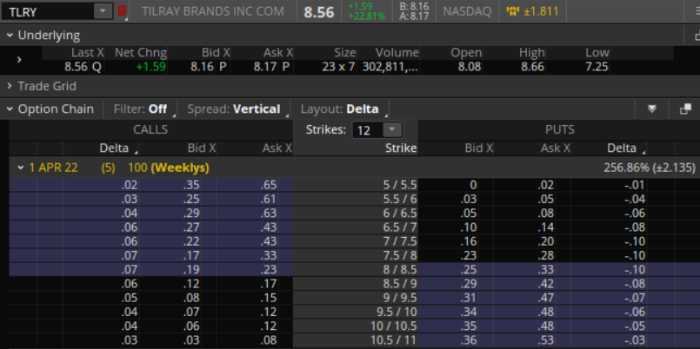## What to be aware of with Options Spreads?

While achieving delta neutrality can be exciting, sometimes doing so comes at a cost. The good news is that option spreads can be a better way to hedge delta. Let's break it down:

• No margin necessary
• Time decay is hedged (super minimal)
• Risk is limited
• Credit spreads allow the ability to profit from time decay

## Vega Neutral Options Strategy | Shorting Vega

Vega is a measure of an option's sensitivity to changes in volatility. When you short vega, you are betting that the underlying security's implied volatility will decrease in the future. This gives options traders the ability to short vega.

Since options decay from time and implied volatility decreases, it is possible to apply similar strategies looking at the greek vega. A vega neutral strategy involves having a vega neutral position using the same tactics to develop delta neutral positions.